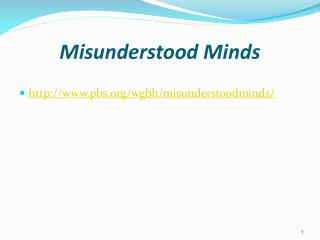DownloadDownload PresentationMisunderstood Minds

# Misunderstood Minds

Télécharger la présentation## Misunderstood Minds

- - - - - - - - - - - - - - - - - - - - - - - - - - - E N D - - - - - - - - - - - - - - - - - - - - - - - - - - -
##### Presentation Transcript

1. Misunderstood Minds • http://www.pbs.org/wgbh/misunderstoodminds/

2. Basics of Mathematics • Mathematics is often thought of as a subject that a student either understands or doesn't, with little in between …

3. Wide Variety Skills and Concepts • Mathematics encompasses a wide variety of skills and concepts. • These skills and concepts are related and often build on one another… • It is possible to master some and still struggle with others.

4. Success in Different Areas A child may have difficulty with basic multiplication facts but may be successful in another area, such as geometry… A student may have some areas of relative strength and others of real vulnerability…

5. Brain Research • Researchers have examined aspects of the brain that are involved when children think with numbers. ..

6. Neurodevelopmental Functions • Memory, • Language, • Attention, • Temporal-sequential ordering, • Higher-order cognition, and • Spatial ordering

7. Process Ongoing • These components become part of an ongoing process in which children constantly integrate new concepts and procedural skills as they solve more advanced math problems.

8. Brain Functions Need to Work Together • Memory to recall rules and formulas and recognize patterns • Language to understand vocabulary, instructions, and explain their thinking • Sequential ordering to solve multi-step problems and use procedures…

9. Brain Functions Need to Work Together • Spatial ordering is important to recognize symbols and deal with geometric forms… • Higher-order cognition helps children to review alternative strategies while solving problems, • to monitor their thinking, • to assess the reasonableness of their answers, • and to transfer and apply learned skills to new problems. • These brain functions often need to operate simultaneously.

10. Try This Problem • Multistep problem from Misunderstood Minds

11. Big Ideas • Math is cumulative • Competence in math draws on more than just the ability to calculate answers correctly

12. Math Encompasses • Problem Solving • Communicating About Mathematical Concepts • Reasoning and Establishing Proof • Representing Information in Different Forms • Making Connections in the Classroom, and Later in the Workplace

13. Developmental Variation and Learning Disorders • Dr. Levine outlines many of these milestones for four age groups, pre-school through grade 12 in his book.

14. Pre-school - Kindergarten • During this stage, children should begin to: count aloud • compute the number of objects in a group • understand that a particular number of objects has a fixed value despite the size or nature of those objects • understand relative size and be able to sort objects by size and shape • follow a sequence of two- and three-step commands • be able to perform simple addition and subtraction computations

15. Grades One to Three • During this stage, children should: begin to perform simple addition and subtraction computations efficiently • Master basic math facts (such as, 3 + 2 = 5) • Recognize and respond accurately to mathematical signs • Begin to grasp the concept of multiplication (grade three) • Understand the notion of measurement and be able to apply this understanding • Improve their concepts of time and money

16. Grades Four to Seven • During this stage, children should: recall basic mathematical facts, including multiplication tables, with ease • Become competent with fractions, decimals, and percentages • Begin to understand the relationships among fractions, decimals, and percentages • Develop facility with word problems • Be adept at estimating quantities and rounding off numbers • Develop basic computer skills

17. Grades Eight to Twelve • During this stage, children should be able to: employ an increasingly high level of abstract, symbolic thinking • Perceive relationships and make translations among decimals, fractions, and percentages • Deal easily with a wide array of equations, formulae, and proofs • Explain and illustrate mathematical concepts, rather than simply apply them • Plan and self-monitor during multi-step problem solving • use calculators and computers with facility

18. Principles and Standards for School Mathematics • http://standards.nctm.org/

19. Connecticut Scope and Sequence Number Sense Operations Estimation Ratio, Proportion and Percent Measurement Spatial Relations and Geometry Probability and Statistics Patterns Algebra and Functions Discrete Mathematics NCTM Content Standards Numbers and Operations Algebra Data Analysis and Probability Geometry Measurement NCTM Process Standards Problem Solving Reasoning and Proof Connections Communication Representation NCTM Standards Compared to Connecticut Scope and Sequence

20. http://www.nctm.org http://www.sde.ct.gov/sde/cwp/view.asp?a=2618&q=320872 NCTM and CT Scope and Sequence